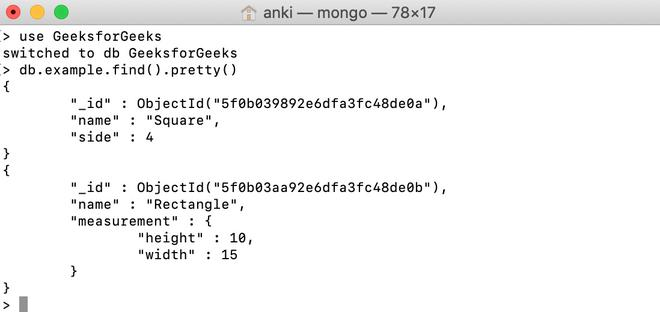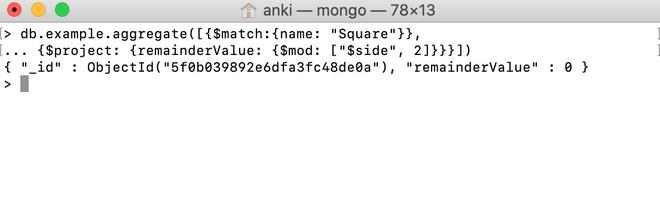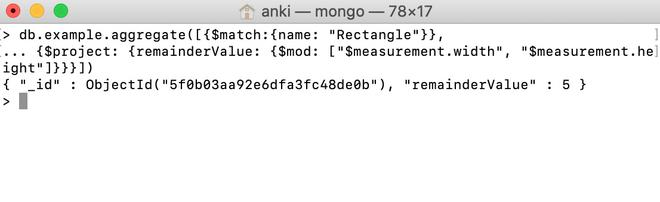# MongoDB \$mod Operator

• Last Updated : 28 Jul, 2020

MongoDB provides different types of arithmetic expression operators that are used in the aggregation pipeline stages and \$mod operator is one of them. This operator is used to divide one number by another number and return the remainder.

Syntax:

```{ \$mod: [ <expression1>, <expression2> ] }
```

Here, in this operator, the arguments passed in an array. It takes two arguments, the first argument is the dividend and the second argument is the divisor.  The arguments must be a valid expression until it resolves to a number.

Examples:

In the following examples, we are working with:

Database: GeeksforGeeks

Collection: example

Document: two documents that contain the details of the shapes in the form of field-value pairs.Finding reminder using \$mod operator:

In this example, we are going to find the remainder by dividing the value(i.e., 4) of the side field by 2 using \$mod operator.

```db.example.aggregate([{\$match:{name: "Square"}},
... {\$project: {remainderValue: {\$mod: ["\$side", 2]}}}])
```Finding reminder in the embedded document using \$mod operator:

In this example, we are going to find the remainder by dividing the value(i.e., 15) of the measurement.width field by the value(i.e., 10) of measurement.height field using \$mod operator.

```db.example.aggregate([{\$match:{name: "Rectangle"}},
... {\$project: {remainderValue: {\$mod: ["\$measurement.width",
"\$measurement.height"]}}}])
```My Personal Notes arrow_drop_up